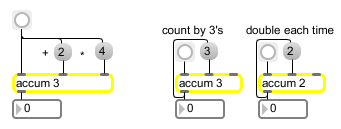# accum

Store, add to, and multiply a number

## Description

accum stores a value (int or float) and adds and/or multiplies into it. If the argument is an integer, the multiplication is still done in floating-point but is then converted back.

## Arguments

Name Type Opt Description
initial-value/number-format int or float opt Sets the initial value stored in accum. An argument with a decimal point causes the value to be stored as a float.

## Messages

 bang In left inlet: Outputs the value currently stored in accum. int input [int] In left inlet: Replaces the value stored in accum, and sends the new value out the outlet. (inlet1) add-to-value [int] In middle inlet: The number is added to the stored value, without triggering output. (inlet2) multiply-by-value [float] In right inlet: The stored value is multiplied by the input, without triggering output. float input [float] In left and middle inlet: Converted to int, unless accum has a float argument. In right inlet: Multiplication is done with floats, even if the value is stored as an int. set input [int] In left inlet: The word set, followed by a number, sets the stored value to that number, without triggering output.

## Output

float: Floats are output only if there is an argument with a decimal point.
int: The value currently held by accum.

## ExamplesAdd to and/or multiply a stored value....... Used here to increment by different amounts